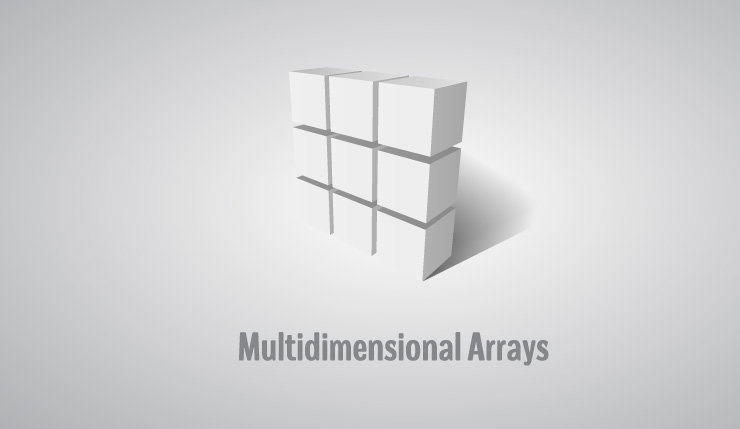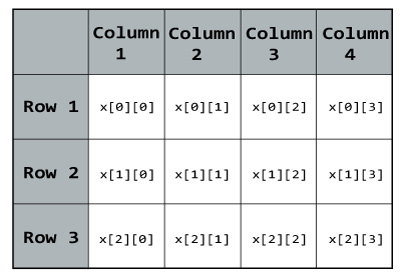# C++ Multidimensional Arrays

In this article, you'll learn about multi-dimensional arrays in C++. More specifically, how to declare them, access them and use them efficiently in your program.In C++, you can create an array of an array known as multi-dimensional array. For example:

`int x;`

Here, x is a two dimensional array. It can hold a maximum of 12 elements.

You can think this array as table with 3 rows and each row has 4 columns as shown below.Three dimensional array also works in a similar way. For example:

`float x;`

This array x can hold a maximum of 24 elements. You can think this example as: Each of the 2 elements can hold 4 elements, which makes 8 elements and each of those 8 elements can hold 3 elements. Hence, total number of elements this array can hold is 24.

## Multidimensional Array Initialisation

You can initialise a multidimensional array in more than one way.

### Initialisation of two dimensional array

`int test = {2, 4, -5, 9, 0, 9};`

Better way to initialise this array with same array elements as above.

`int  test = { {2, 4, 5}, {9, 0 0}};`

### Initialisation of three dimensional array

```int test = {3, 4, 2, 3, 0, -3, 9, 11, 23, 12, 23,
2, 13, 4, 56, 3, 5, 9, 3, 5, 5, 1, 4, 9};
```

Better way to initialise this array with same elements as above.

```int test = {
{ {3, 4, 2, 3}, {0, -3, 9, 11}, {23, 12, 23, 2} },
{ {13, 4, 56, 3}, {5, 9, 3, 5}, {3, 1, 4, 9} }
};
```

## Example 1: Two Dimensional Array

C++ Program to display all elements of an initialised two dimensional array.

``````#include <iostream>
using namespace std;

int main()
{
int test =
{
{2, -5},
{4, 0},
{9, 1}
};

// Accessing two dimensional array using
// nested for loops
for(int i = 0; i < 3; ++i)
{
for(int j = 0; j < 2; ++j)
{
cout<< "test[" << i << "][" << j << "] = " << test[i][j] << endl;
}
}

return 0;
}``````

Output

```test = 2
test = -5
test = 4
test = 0
test = 9
test = 1
```

## Example 2: Two Dimensional Array

C++ Program to store temperature of two different cities for a week and display it.

``````#include <iostream>
using namespace std;

const int CITY = 2;
const int WEEK = 7;

int main()
{
int temperature[CITY][WEEK];

cout << "Enter all temperature for a week of first city and then second city. \n";

// Inserting the values into the temperature array
for (int i = 0; i < CITY; ++i)
{
for(int j = 0; j < WEEK; ++j)
{
cout << "City " << i + 1 << ", Day " << j + 1 << " : ";
cin >> temperature[i][j];
}
}

cout << "\n\nDisplaying Values:\n";

// Accessing the values from the temperature array
for (int i = 0; i < CITY; ++i)
{
for(int j = 0; j < WEEK; ++j)
{
cout << "City " << i + 1 << ", Day " << j + 1 << " = " << temperature[i][j] << endl;
}
}

return 0;
}
``````

Output

```Enter all temperature for a week of first city and then second city.
City 1, Day 1 : 32
City 1, Day 2 : 33
City 1, Day 3 : 32
City 1, Day 4 : 34
City 1, Day 5 : 35
City 1, Day 6 : 36
City 1, Day 7 : 38
City 2, Day 1 : 23
City 2, Day 2 : 24
City 2, Day 3 : 26
City 2, Day 4 : 22
City 2, Day 5 : 29
City 2, Day 6 : 27
City 2, Day 7 : 23

Displaying Values:
City 1, Day 1 = 32
City 1, Day 2 = 33
City 1, Day 3 = 32
City 1, Day 4 = 34
City 1, Day 5 = 35
City 1, Day 6 = 36
City 1, Day 7 = 38
City 2, Day 1 = 23
City 2, Day 2 = 24
City 2, Day 3 = 26
City 2, Day 4 = 22
City 2, Day 5 = 29
City 2, Day 6 = 27
City 2, Day 7 = 23
```

### Example 3: Three Dimensional Array

C++ Program to Store value entered by user in three dimensional array and display it.

``````#include <iostream>
using namespace std;

int main()
{
// This array can store upto 12 elements (2x3x2)
int test;

cout << "Enter 12 values: \n";

// Inserting the values into the test array
// using 3 nested for loops.
for(int i = 0; i < 2; ++i)
{
for (int j = 0; j < 3; ++j)
{
for(int k = 0; k < 2; ++k )
{
cin >> test[i][j][k];
}
}
}

cout<<"\nDisplaying Value stored:"<<endl;

// Displaying the values with proper index.
for(int i = 0; i < 2; ++i)
{
for (int j = 0; j < 3; ++j)
{
for(int k = 0; k < 2; ++k)
{
cout << "test[" << i << "][" << j << "][" << k << "] = " << test[i][j][k] << endl;
}
}
}

return 0;
}``````

Output

```Enter 12 values:
1
2
3
4
5
6
7
8
9
10
11
12

Displaying Value stored:
test = 1
test = 2
test = 3
test = 4
test = 5
test = 6
test = 7
test = 8
test = 9
test = 10
test = 11
test = 12
```

As the number of dimension increases, the complexity also increases tremendously although the concept is quite similar.